# Increasing functions, decreasing functions

## Increasing function

Definition: a Functionis called increasing on some set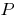if a greater argument value from this set corresponds to the greater value of the function.— increasing, if for any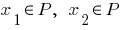### Properties an increasing function of

1. If the functionis increasing on some set, the greater the value of the function there corresponds a large value of the argument from this set
2.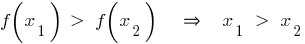3. The sum of several growing on this set of functions is an increasing function on this set.
4. If the functionis increasing, then reverse to it function also increases.
5. If the composed function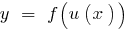a function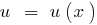increases the function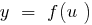increases, the functionincreases. The result of successive application of two increasing functions is an increasing function.
6. The result of consecutive application of increasing and decreasing functions a function is decreasing.
7. Growing on any given set the function of each acquires its value only in one point from this set.
8.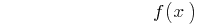— increasing function— increasing function

### A sign of increasing functions

Ifat each point of the interval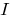, the functionis increasing on this interval.

### Examples of functions are increasing in all scope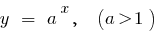## Descending function

Definition: a Functionis called decreasing on some setif a greater argument value from this set corresponds to the greater value of the function.— if for any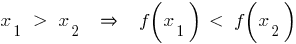### The properties of a decreasing function

1. If the functionspadano a lot, the more the value of the function corresponds to the least value of the argument from this set
2.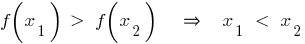3. The amount of multiple descending on this set of functions is a decreasing function on this set.
4. If the functionis decreasing, then the reverse to it function also decreases.
5. If the composed functionis the functiondecreasing and the functionis decreasing, then the functiondecreases. The result of successive application of two decreasing functions is an increasing function.
6. The result of consecutive application of increasing and decreasing functions a function is decreasing.
7. Descending on any given set the function of each acquires its value only in one point from this set.
8.— decreasing function— decreasing function

### Signs of decreasing function

If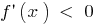at each point of the interval, the functionis decreasing on this interval.

### Examples of features falling at the entire scope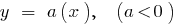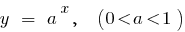Tags:
Chapter:
Versions in other languages:
Share with friends: